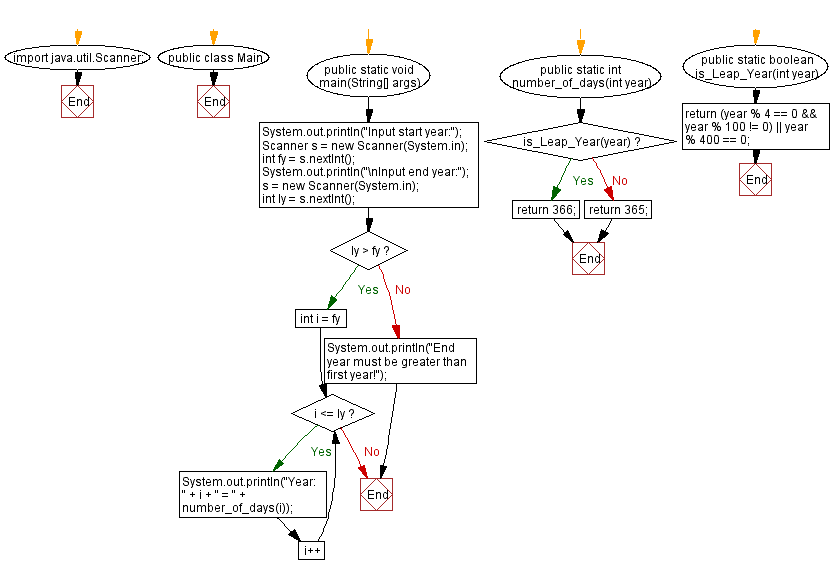﻿ Java - Count the number of days between two given years

# Java: Count the number of days between two given years

## Java DateTime, Calendar: Exercise-46 with Solution

Write a Java program to count the number of days between two years.

Sample Solution:

Java Code:

``````import java.util.Scanner;
public class test {
public static void main(String[] args) {
System.out.println("Input start year:");
Scanner s = new Scanner(System.in);
int fy = s.nextInt();
System.out.println("\nInput end year:");
s = new Scanner(System.in);
int ly = s.nextInt();
if (ly > fy) {
for (int i = fy; i <= ly; i++) {
System.out.println("Year: " + i + " = " + number_of_days(i));
}
} else {
System.out.println("End year must be greater than first year!");
}
}
public static int number_of_days(int year) {
if (is_Leap_Year(year)) return 366;
else return 365;
}
public static boolean is_Leap_Year(int year) {
return (year % 4 == 0 && year % 100 != 0) || year % 400 == 0;
}
}
```
```

Sample Output:

```Input start year:
2010

Input end year:
2022
Year: 2010 = 365
Year: 2011 = 365
Year: 2012 = 366
Year: 2013 = 365
Year: 2014 = 365
Year: 2015 = 365
Year: 2016 = 366
Year: 2017 = 365
Year: 2018 = 365
Year: 2019 = 365
Year: 2020 = 366
Year: 2021 = 365
Year: 2022 = 365
```

Flowchart:Java Code Editor:

Improve this sample solution and post your code through Disqus

What is the difficulty level of this exercise?

Test your Programming skills with w3resource's quiz.

﻿